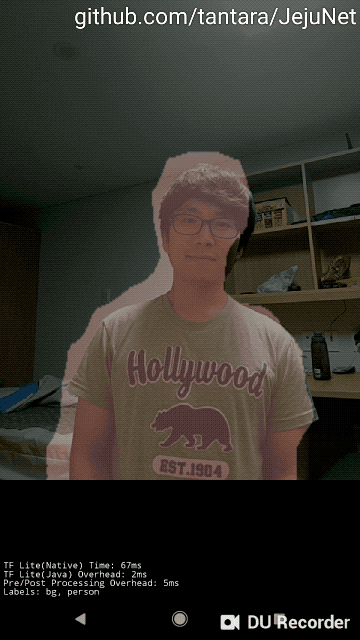生产与学术

By Lart, 2019-01-08https://github.com/lartpang/DHSNet-PyTorch/blob/master/converter.ipynb

这两天

 `````` 1 2 3 4 5 6 7 8 9 10 11 12 13 14 15 16 17 18 19 20 21 22 23 24 25 `````` ``````def squeezenet1_1(pretrained=False, **kwargs): r"""SqueezeNet 1.1 model from the `official SqueezeNet repo `_. SqueezeNet 1.1 has 2.4x less computation and slightly fewer parameters than SqueezeNet 1.0, without sacrificing accuracy. Args: pretrained (bool): If True, returns a model pre-trained on ImageNet """ model = SqueezeNet(version=1.1, **kwargs) if pretrained: model.load_state_dict(model_zoo.load_url(model_urls['squeezenet1_1'])) return model # Get pretrained squeezenet model torch_model = squeezenet1_1(True) from torch.autograd import Variable batch_size = 1 # just a random number # Input to the model x = Variable(torch.randn(batch_size, 3, 224, 224), requires_grad=True) # Export the model torch_out = torch.onnx._export( torch_model, # model being run x, # model input (or a tuple for multiple inputs) "squeezenet.onnx", # where to save the model (can be a file or file-like object) export_params=True) # store the trained parameter weights inside the model file``````

 ``````1 2 3 4 5 6 7 8 9 `````` ``````# Create the super-resolution model by using the above model definition. torch_model = SuperResolutionNet(upscale_factor=3) # Load pretrained model weights model_url = 'https://s3.amazonaws.com/pytorch/test_data/export/superres_epoch100-44c6958e.pth' batch_size = 1 # just a random number # Initialize model with the pretrained weights torch_model.load_state_dict(model_zoo.load_url(model_url)) # set the train mode to false since we will only run the forward pass. torch_model.train(False)``````

导出整体模型

 ``````1 2 `````` ``````filename_opti = ('%s/model-best.pth' % check_root_model) torch.save(model, filename_opti)``````

 `````` 1 2 3 4 5 6 7 8 9 10 `````` ``````IMG_SIZE = 224 TMP_ONNX = 'cache/onnx/DHSNet.onnx' MODEL_PATH = 'cache/opti/total-opti-current.pth' # Convert to ONNX once model = torch.load(MODEL_PATH).cuda() model.train(False) x = Variable(torch.randn(1, 3, 224, 224), requires_grad=True).cuda() torch_out = torch.onnx._export(model, x, TMP_ONNX, export_params=True)``````

caffe2模型转换

 ``````1 2 3 4 5 `````` ``````# Let's also save the init_net and predict_net to a file that we will later use for running them on mobile with open('./cache/model_mobile/init_net.pb', "wb") as fopen: fopen.write(init_net.SerializeToString()) with open('./cache/model_mobile/predict_net.pb', "wb") as fopen: fopen.write(predict_net.SerializeToString())``````

预处理的补充

 ``````1 2 3 4 5 6 7 8 9 `````` ``````# load the resized image and convert it to Ybr format mean = np.array([0.485, 0.456, 0.406]) std = np.array([0.229, 0.224, 0.225]) img = Image.open("./data/ILSVRC2012_test_00000004_224x224.jpg") img = np.array(img) img = img.astype(np.float64) / 255 img -= mean img /= std img = img.transpose(2, 0, 1)``````

安卓的尝试

AiCamera

• 输入有差异: 比赛要求的是若是提交apk, 那么要求可以从相册读取图片, 而例子是从摄像头读取的视频数据流. 虽然也处理的是视频帧, 但是要我们再次补充的内容又多了起来, 还是那句话, android一窍不通.
• 输出有差异: 自我猜测, 比赛为了测评, 输出必然也要输出到相册里, 不然何来测评一说?

JejuNetpickle和onnx的限制

pytorch的`torch.save(model)`保存模型的时候, 模型架构的代码里不能使用一些特殊的构建形式, R3Net的ResNeXt结构就用了, 主要是一些lambda结构, 虽然不是太清楚, 但是一般的搭建手段都是可以的.

onnx对于pytorch的支持的操作, 在我的转化中, 主要是最大池化和上采样的问题, 前者可以修改`ceil_mode``False`, 后者则建议修改为转置卷积, 避免不必要的麻烦. 可见“导出整体模型”小节的描述.